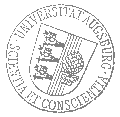# Universität AugsburgInstitut für MathematikOberseminar Stochastik

Professor Dr. Alessandra Bianchi

spricht am

Mittwoch, 5. Juli 2023

um

16:00 Uhr

im

Raum 1010 (L1)

über das Thema:

## »Limit Theorems for Exponential Random Graphs«

 Abstract: Exponential Random Graphs are defined through probabilistic ensembles with one or more adjustable parameters. They can be seen as a generalization of the classical Erdos Renyi random graph, obtained by defining a tilted probability measure that is proportional to the densities of certain given finite subgraphs. In this talk we will focus on the edge-triangle model, that is a two-parameter family of exponential random graphs in which dependence between edges is introduced through triangles. Borrowing tools from statistical mechanics, together with large deviations techniques, we will characterize the limiting behavior of the edge density for all parameters in the so-called replica symmetric regime, where a complete characterization of the phase diagram of the model is accessible. First, we determine the asymptotic distribution of this quantity, as the graph size tends to infinity, in the various phases. Then we study the fluctuations of the edge density around its average value off the critical curve and formulate conjectures about the behavior at criticality based on the analysis of a mean-field approximation of the model. Joint work with Francesca Collet and Elena Magnanini.

 Hierzu ergeht herzliche Einladung. Prof. Dr. Stefan Großkinsky

[Impressum]      [Datenschutz]      wwwadm@math.uni-augsburg.de,     Do 15-Jun-2023 10:18:05 MESZ